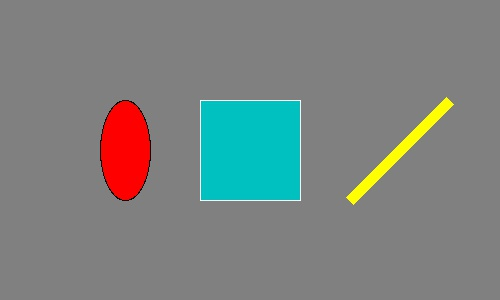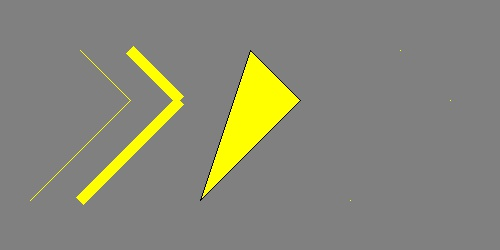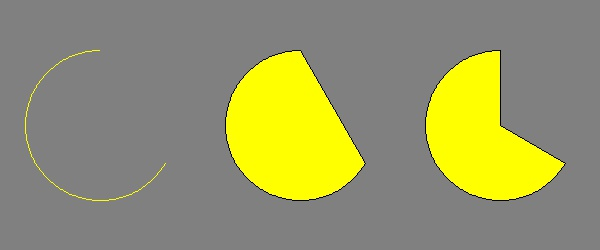# Draw circle, rectangle, line, etc. with Python, Pillow

Posted: 2019-05-14 / Tags: Python, Pillow, Image Processing

`ImageDraw` module of the Python image processing library Pillow (PIL) provides a number of methods for drawing figures such as circle, square, and straight line.

See the following article for the installation and basic usage of Pillow (PIL).

## Flow of drawing figures

### Create Draw Object

Prepare an `Image` object of a background image (image for drawing a figure) and use it to create a `Draw` object. Don't forget to import `Image` and `ImageDraw`.

``````from PIL import Image, ImageDraw

im = Image.new('RGB', (500, 300), (128, 128, 128))
draw = ImageDraw.Draw(im)
``````

Here, create a solid image with `Image.new()`. The mode, size, and fill color are specified in parameters.

### Draw a shape with the drawing method

Call the drawing method from the `Draw` object to draw a figure.

Draw an ellipse, a rectangle, and a straight line as an example. The parameters will be described later.

``````draw.ellipse((100, 100, 150, 200), fill=(255, 0, 0), outline=(0, 0, 0))
draw.rectangle((200, 100, 300, 200), fill=(0, 192, 192), outline=(255, 255, 255))
draw.line((350, 200, 450, 100), fill=(255, 255, 0), width=10)

im.save('data/dst/pillow_imagedraw.jpg', quality=95)
``````## Drawing method

### Common parameters

Although the method differs depending on the method, the following parameters are common.

#### xy

Set a rectangular area to draw a figure.

Specify in one of the following formats:

• `(((Upper left x coordinate, upper left y coordinate), (lower right x coordinate, lower right y coordinate))`
• `(Upper left x coordinate, upper left y coordinate, lower right x coordinate, lower right y coordinate)`

In `line()`, `polygon()`, and `point()`, multiple coordinates are specified instead of two points representing a rectangular area.

• `(x1, y1, x2, y2, x3, y3...)`
• `((x1, y1), (x2, y2), (x3, y3)...)`

`line()` draws a straight line connecting each point, `polygon()` draws a polygon where each point is connected , and `point()` draws a point of 1 pixel at each point.

#### fill

Set the color to fill the shape.

The specification format differs depending on the mode of the image (`Image` object).

• `RGB`: Set each color value (0-255) in the form of `(R, G, B)`
• `L` (Grayscale): Set a value (0-255) as an integer

The default is `None` (no fill).

#### outline

Set the border color of the figure.

The specification format of color is the same as `fill` above. The default is `None` (no border).

As of version `4.4.0`, there is no option to set the line width (line thickness) other than `line()`.

### Method example

See the official document for details.

#### Ellipse, rectangle

• Ellipse (Circle): `ellipse(xy, fill, outline)`
• Rectangle (Square): `rectangle(xy, fill, outline)`

`ellipse()` draws an ellipse tangent to the rectangular area specified by the argument `xy`. Specifying a square results in a true circle.

The output results are as shown in the above example.

#### Line, polygon, point

• Line: `line(xy, fill, width)`
• `xy`
• Set multiple coordinates of two or more points as `((x1, y1), (x2, y2), (x3, y3)...)`.
• Lines connecting each point is drawn.
• `width`: Line width (line thickness)
• Note that if you make the line width thicker with `width`, specifying 3 points or more with `xy` will make the connection look unattractive.
• Polygon: `polygon(xy, fill, outline)`
• `xy`
• Set multiple coordinates of three or more points as `((x1, y1), (x2, y2), (x3, y3)...)`.
• A polygon in which each point is connected is drawn.
• Point : `point(xy, fill)`
• `xy`
• Set multiple coordinates of one or more points as `((x1, y1), (x2, y2), (x3, y3)...)`.
• One pixel point is drawn for each points.

The example of lines (`line()`), polygon (`polygon()`), point (`point()`) is as follows. Since the point is 1 pixel, it is hard to see but it is drawn on the right side.

``````im = Image.new('RGB', (500, 250), (128, 128, 128))
draw = ImageDraw.Draw(im)

draw.line(((30, 200), (130, 100), (80, 50)), fill=(255, 255, 0))
draw.line(((80, 200), (180, 100), (130, 50)), fill=(255, 255, 0), width=10)
draw.polygon(((200, 200), (300, 100), (250, 50)), fill=(255, 255, 0), outline=(0, 0, 0))
draw.point(((350, 200), (450, 100), (400, 50)), fill=(255, 255, 0))
``````#### Arc, chord, pie

An arc, a chord (bow), and a pie touching the rectangular area specified by the argument `xy` are drawn.

• Arc: `arc(xy, start, end, fill)`
• `start`, `end`
• Set the angle of the arc in degrees.
• 0 degrees is the direction of 3 o'clock. clockwise.
• Chord (bow) : `chord(xy, start, end, fill, outline)`
• The start and end points of the arc are connected by a straight line.
• Pie : `pieslice(xy, start, end, fill, outline)`
• The start and end points of the arc are connected by a straight line to the center of the circle.

Example of arc (`arc()`), chord (`chord()`), pie (`pieslice()`) is as follows.

``````im = Image.new('RGB', (600, 250), (128, 128, 128))
draw = ImageDraw.Draw(im)

draw.arc((25, 50, 175, 200), start=30, end=270, fill=(255, 255, 0))
draw.chord((225, 50, 375, 200), start=30, end=270, fill=(255, 255, 0), outline=(0, 0, 0))
draw.pieslice((425, 50, 575, 200), start=30, end=270, fill=(255, 255, 0), outline=(0, 0, 0))
``````## Draw on image

In the previous examples, figures are drawn on the solid image generated by `Image.new()`. If an existing image file is read by `Image.open()`, it can be drawn on it.

``````im = Image.open('data/src/lena.jpg')
draw = ImageDraw.Draw(im)

draw.pieslice((15, 50, 140, 175), start=30, end=330, fill=(255, 255, 0))
``````